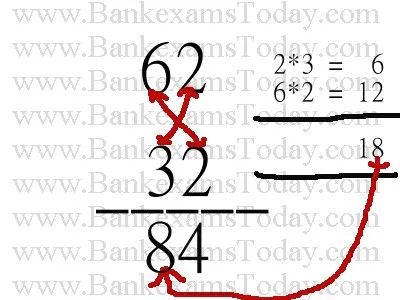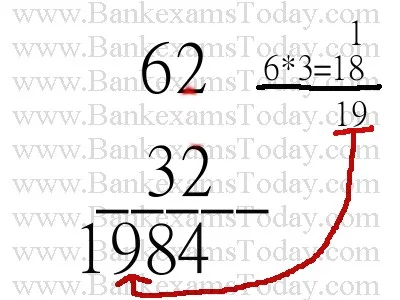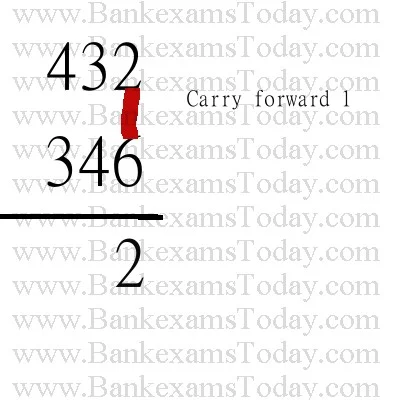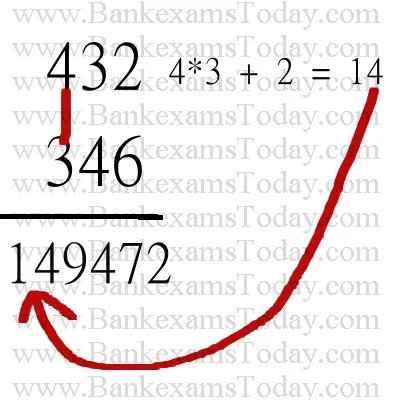New Student User - Use Code HELLO

# 3 Steps Multiplication Trick

In the series of providing quantitative shortcuts and tricks, today I come up with multiplication trick.

While doing multiplication of a two digit number with another two digit number, we take at least 6 steps. Try yourself. Multiply 62 with 32.

Now let's do this with a trick

## Step 1

First step is same as the conventional method, here we multiply 2 with 2.## Step 2

This is an interesting step. Now multiply last digit first value and first digit of second value and vice-versa. Then we add outcomes. But we need the last number that is 8 here.## Step 3

This is the last step, in this step we do multiplication ten's digit of both value and add the remainder from previous calculation. That's it, we completed the calculation in 3 steps instead of six steps.We can use this method for multiplication of three or even four-digit numbers but time management is really important in IBPS exam and other recruitment exams so, for longer calculations, estimation is the best trick. I will post an article about how to do long calculations using estimation and result is 95% accurate which is enough to arrive at the answer.

Update 06-09-2013

As two of the readers namely Rahul and Ansh have requested me to use this technique in longer calculations multiplications. I am updating this article.

## Multiplication of 3 digit numbers

In this example I will multiply 432 with 346. Now the 3 step multiplication method will become 5 step. This method can be used for 4 and even 5 digit numbers but as in bank exams there is lack of time available for calculations I recommend you to use the approximation for long calculations.

### Step 1### Step 2### Step 3### Step 4### Step 5In case you find any difficulty to understand the above multiplication method then ask your question in the comments. I will try to answer every query asap.

Further, read 10 Maths Tricks here